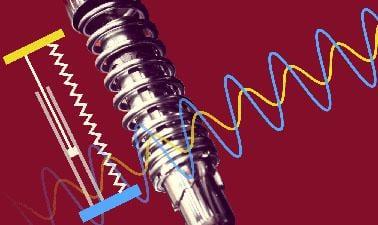# Introduction to Differential Equations

Scientists and engineers understand the world through differential equations. You can too.### There is one session available:

39,134 already enrolled! After a course session ends, it will be archived.
Starts Jun 2
Ends Sep 1
Estimated 14 weeks
3–6 hours per week
Instructor-paced
Instructor-led on a course schedule

How do you design:

• A boat that doesn’t tip over as it bobs in the water?
• The suspension system of a car for a smooth ride?
• Circuits that tune to the correct frequencies in a cell phone?

How do you model:

• The growth of antibiotic resistant bacteria?
• Gene expression?

Differential equations are the language of the models we use to describe the world around us. In this mathematics course, we will explore temperature, spring systems, circuits, population growth, and biological cell motion to illustrate how differential equations can be used to model nearly everything in the world around us.

We will develop the mathematical tools needed to solve linear differential equations. In the case of nonlinear differential equations, we will employ graphical methods and approximation to understand solutions.

The five modules in this seriesare being offered as an XSeries on edX. Please visit the Differential EquationsXSeries Program Pageto learn more and to enroll in the modules.

### At a glance

• Institution: MITx
• Subject: Math
• Level: Introductory
• Prerequisites:

Single variable calculus

# What you'll learn

Skip What you'll learn
• Use linear differential equations to model physical systems using the input / system response paradigm.
• Solve linear differential equations with constant coefficients.
• Gain intuition for the behavior of a damped harmonic oscillator.
• Understand solutions to nonlinear differential equations using qualitative methods.

# Syllabus

Skip Syllabus

Unit 1

1. Introduction to differential equations and modeling
2. Complex numbers
3. Solving first order linear differential equations

Unit 2

1. The complex exponential
2. Sinusoids
3. Higher order linear differential equations
4. Characteristic polynomial

Unit 3

1. Harmonic oscillators
2. Operators
3. Complex replacement
4. Resonance

Unit 4

1. Graphical methods and nonlinear differential equations
2. Autonomous equations
3. Numerical methods